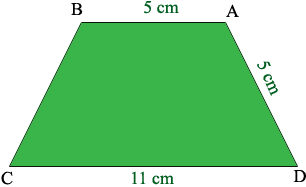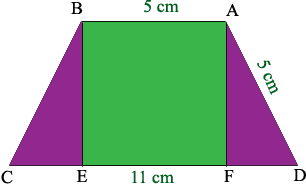SEARCH HOMEMath Central Quandaries & QueriesQuestion from lyjah, a student: what is parallel sides of an isosceles trapezoid measure 5cm and 11cm long.and oneof the other sides also measures s5cn long what of the isosceles trapeziodLyjah,

I'm not sure that I am interpreting your problem correctly but I think you have an isosceles trapezoid with the parallel sides of length 11cm and 5 cm and one of the remaining two sides has length 5 cm also. Do you want to find the height of the trapezoid? If so I would proceed as follows.

Draw a diagram.Since the trapezoid is isosceles the length of the side BC is 5 cm also. I then drew a vertical line from A to the base and from B to the base.What do you know about triangles AFD and BCE? What is the length of FD? What does Pythagoras tell you about the length of AF, the height of the trapezoid?

PennyMath Central is supported by the University of Regina and The Pacific Institute for the Mathematical Sciences.